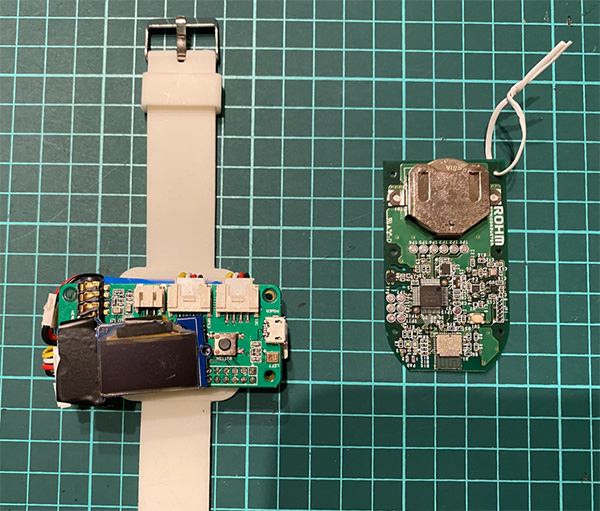## 用Raspberry Pi和传感器制作“可自动营造舒适空间的装置” 第三部分## 本部分所需部件

### Raspberry Pi 3 B+ 或 Raspberry Pi 4 Model BRaspberry Pi 3 B+Raspberry Pi 4 Model B

### 罗姆SensorMedal（SensorMedal-EVK-002）### 热释电红外线传感器 (SB412A)### Raspberry Pi用液晶显示器## 本部分的流程

1. 给Raspberry Pi连接人体传感器
2. 添加液晶显示器
3. 总结

## 1. 给Raspberry Pi连接人体传感器

 编号 检测功能 检测后希望具备的功能 5 检测坐在椅子上的时间 检测到坐的时间太久（久坐不动），督促主人站起来活动活动传感器侧（从照片左边开始） Raspberry Pi侧 ＋（黄色） 5V OUT（红色） GPIO13 ー（黑色） GND[human.py]

```import RPi.GPIO as GPIO
from time import sleep

human_pin = 13
GPIO.setmode(GPIO.BCM)
GPIO.setup(human_pin, GPIO.IN)

try:
while True:
human = GPIO.input(human_pin)
print(human)
sleep(1)

except KeyboardInterrupt:
pass

GPIO.cleanup()
```## 2. 添加液晶显示器https://github.com/pimoroni/displayotron

Dot3000和DotHat都有类似的功能，但是这次我使用的是DotHat，所以我们会使用DotHat对应的库。这里有一些示例程序，您可以尝试运行一下。

```\$ curl -sS get.pimoroni.com/displayotron | bash
\$ cd /home/pi/Pimoroni/displayotron/dothat
\$ python3 basic/hello_world.py
\$ python3 basic/backlight.py
```

[ble_lcd.py]

```#!/usr/bin/env python3
# coding: utf-8

import dothat.lcd as lcd

from datetime import datetime
from bluepy import btle
from sys import argv
import getpass
from time import sleep

def payval(num, bytes=1, sign=False):
global val
a = 0
for i in range(0, bytes):
a += (256 ** i) * int(val[(num - 2 + i) * 2 : (num - 1 + i) * 2],16)
if sign:
if a >= 2 ** (bytes * 8 - 1):
a -= 2 ** (bytes * 8)
return a

import RPi.GPIO as GPIO
human_pin = 13
GPIO.setmode(GPIO.BCM)
GPIO.setup(human_pin, GPIO.IN)
human_count = 0
human_check = 3

interval = 10 # 工作间隔
scanner = btle.Scanner()

while True:

now = datetime.now()
d = '{0:0>4d}/{1:0>2d}/{2:0>2d}({3})'.format(now.year, now.month, now.day, now.strftime('%a'))
t = '{0:0>2d}:{1:0>2d}:{2:0>2d}'.format(now.hour, now.minute, now.second)
lcd.clear()
lcd.set_cursor_position(0, 0)
lcd.write('PiRemote')
lcd.set_cursor_position(0, 1)
lcd.write('{}'.format(d))
lcd.set_cursor_position(2, 2)
lcd.write('{}'.format(t))

human = GPIO.input(human_pin)
print(human)
if human == 1:
human_count+=1
else:
human_count=0

try:
devices = scanner.scan(interval)
except Exception as e:
print("ERROR",e)
if getpass.getuser() != 'root':
print('使用方法: sudo', argv)
exit()
sleep(interval)
continue

for dev in devices:
isRohmMedal = False
sensors = dict()
for (adtype, desc, val) in dev.getScanData():
print("  %s = %s" % (desc, val))
if desc == 'Short Local Name' and val[0:10] == 'ROHMMedal2':
isRohmMedal = True
if isRohmMedal and desc == 'Manufacturer':

sensors['ID'] = hex(payval(2,2))
sensors['Temperature'] = -45 + 175 * payval(4,2) / 65536
sensors['Humidity'] = 100 * payval(6,2) / 65536
sensors['Illuminance'] = payval(25,2) / 1.2
sensors['Battery Level'] = payval(30)

print('    ID            =',sensors['ID'])
print('    Temperature   =',round(sensors['Temperature'],2),'℃')
print('    Humidity      =',round(sensors['Humidity'],2),'%')
print('    Illuminance   =',round(sensors['Illuminance'],1),'lx')
print('    Battery Level =',sensors['Battery Level'],'%')

'''
for key, value in sorted(sensors.items(), key=lambda x:x):
print('    ',key,'=',value)
'''

temp  = sensors['Temperature']
humid = sensors['Humidity']
if temp > 26 or humid > 60:
temp_msg = "Hot!"
else:

illum = sensors['Illuminance']
if illum < 200:
illum_msg = "Dark!"
else:
illum_msg = "Bright"

if human_count > human_check:
human_msg = "Take Rest"
else:
human_msg = "Work Hard!"

lcd.clear()
lcd.set_cursor_position(0, 0)
lcd.write('Temp: {0:1.0f}C {1:1.0f}% {2}'.format(temp, humid, temp_msg))
lcd.set_cursor_position(0, 1)
lcd.write('Illum: {0:1.0f}Lx {1}'.format(illum,illum_msg))
lcd.set_cursor_position(0, 2)
lcd.write('{0:1.0f} Cnt {1}'.format(human_count, human_msg))

sleep(interval)
```## 3. 总结##### 吉田　显一（Yoshiken）

http://blog.ktrips.net

### 相关文章

1.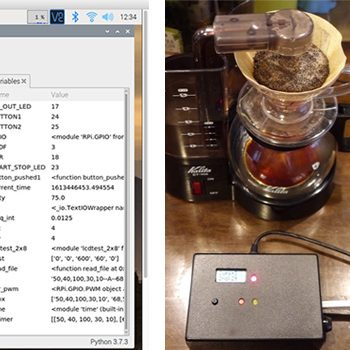#### 使用Raspberry Pi制作咖啡机控制装置 第3部分：使用Python完成控制软件

2.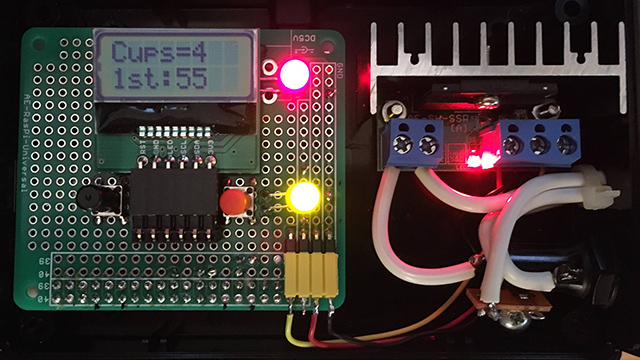#### 使用Raspberry Pi制作咖啡机控制装置 第2部分：硬件制作

3.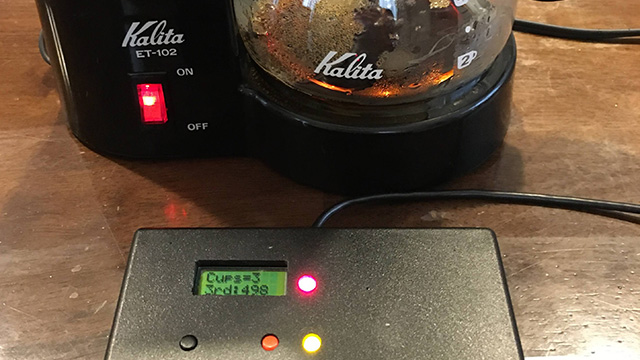#### 使用Raspberry Pi制作咖啡机控制装置 第1部分：装置概况和咖啡萃取

4.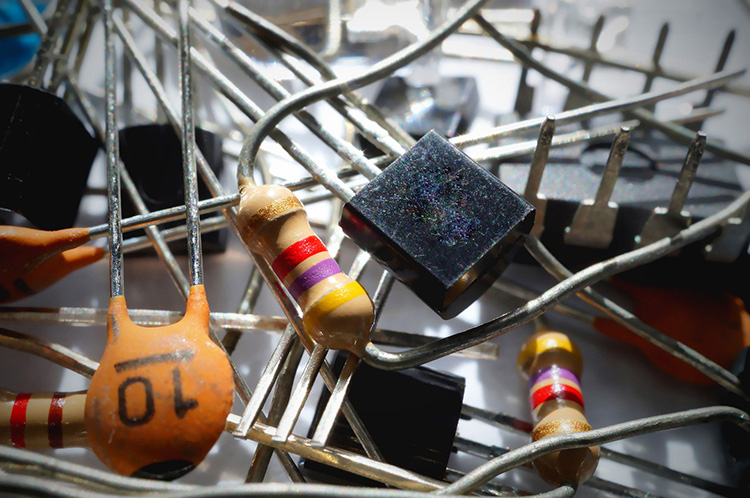#### 数字晶体管是削减元器件、创建简单电路的第一步

5.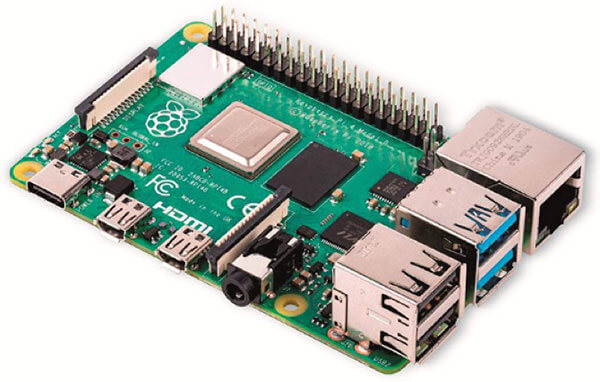#### 从历史到使用方法的全面了解！电子作品创作不可或缺的“Raspberry Pi（树莓派）”究竟是何方神…

6.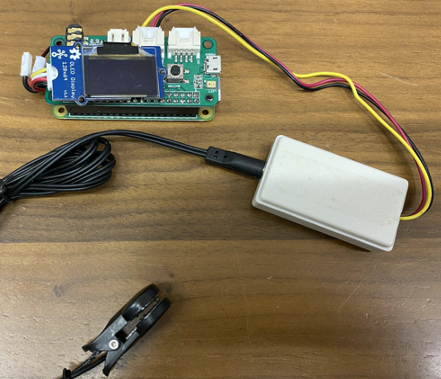#### 一款用Raspberry Pi和传感器制作的可穿戴式小型健康管理设备！第四部分•剧终篇

7.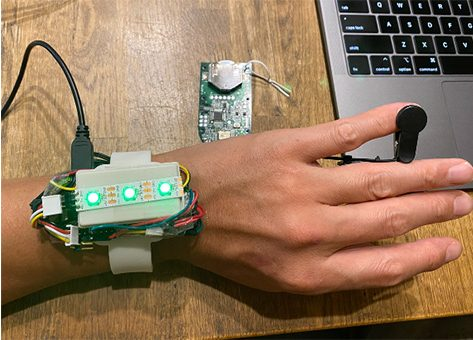#### 一款用Raspberry Pi和传感器制作的可穿戴式小型健康管理设备！第三部分

8.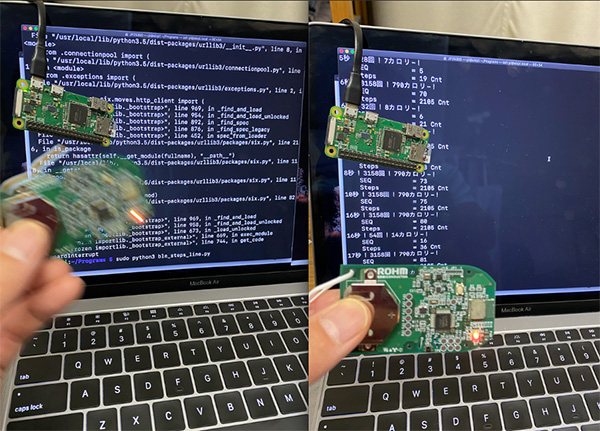#### 一款用Raspberry Pi和传感器制作的可穿戴式小型健康管理设备！第二部分

9.